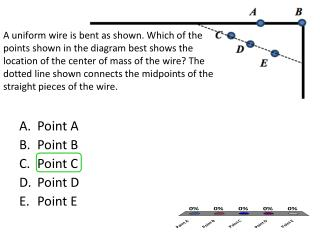DownloadDownload PresentationPoint A Point B Point C Point D Point E

Point A Point B Point C Point D Point E

Télécharger la présentationPoint A Point B Point C Point D Point E

- - - - - - - - - - - - - - - - - - - - - - - - - - - E N D - - - - - - - - - - - - - - - - - - - - - - - - - - -
Presentation Transcript

1. A uniform wire is bent as shown. Which of the points shown in the diagram best shows the location of the center of mass of the wire? The dotted line shown connects the midpoints of the straight pieces of the wire. • Point A • Point B • Point C • Point D • Point E

2. Two discs of radii 8.0 cm and 4.0 cm are made of cardboard and are placed on a flat table as shown. How far from the center of the larger disc is the center of mass of the entire figure located? • 2.0 cm • 2.4 cm • 3.0 cm • 4.0 cm • 6.0 cm • 9.6 cm

3. Three tiny balls of equal mass are placed on a horizontal frictionless surface at the corners of an equilateral triangle (all of the sides are 2 m and all of the angles are 60°). What are the x and y coordinates of the center of mass of the system formed by the three balls? (The y-axis passed through the top ball and the x-axis passed through the bottom balls.) • x=0 m; y=0 m • x=0 m; y=1 m • x=0 m; y=0.58 m • x=0 m; y=0.87 m • x=0.67 m; y=0.67 m • x=0 m; y=0.67 m

4. Four particles are located in a coordinate plane. Their masses and x- and y-coordinates are given in the table (except for the coordinates of particle m4 ). Find those coordinates if the center of mass of all four particles is located at the origin? • x4 = -2 cm; y4 = -7 cm • x4 = 1.5 cm; y4 = -2.5 cm • x4 = 2 cm; y4 = 7 cm • x4 = -1.5 cm; y4 = 2.5 cm • x4 = -2 cm; y4 = 7 cm • x4 = 1.5 cm; y4 = 2.5 cm

5. A large person with mass 2M and a smaller person with mass M are holding onto a massless pole while standing on frictionless ice, as shown below. If the large person pulls himself toward the smaller person along the massless rod, where will they meet? • -3m • -2m • -1m • 0 • 1m • 2m • 3m • None of the above